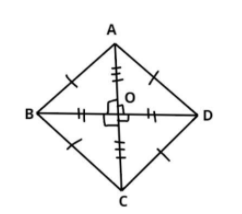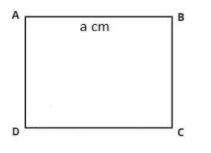QuestionAnswers

# Areas of square and rhombus are equal. A diagonal of a rhombus is twice of its other diagonal. If the area of rhombus is 64 sq.cm find the ratio of perimeter of a square and rhombus.$\left( a \right)\sqrt 3 :1$\left( b \right)2:\sqrt 5$\left( c \right)2:\sqrt 3$$\left( d \right)\sqrt 5 :2$

Hint: In this particular question use the concept that the area of the rhombus is half times the product of the diagonals of the rhombus, and area of the square is equal to the square of its side so use these concepts to reach the solution of the question.

Given data:
Area of rhombus = Area of square = 64 sq. cm.
$\Rightarrow {A_1} = {A_2} = 64$ Sq. cm.
It is also given that a diagonal of a rhombus is twice of its other diagonal.
Let the length of one diagonal is p cm, so the length of the other diagonal is 2p cm.
Now as we all know that the area $\left( {{A_1}} \right)$ of the rhombus is half times the product of the diagonals of the rhombus.
$\Rightarrow {A_1} = \dfrac{{p\left( {2p} \right)}}{2}$ sq.cm.
Now substitute the value and simplify we have,
$\Rightarrow 64 = {p^2}$
$\Rightarrow p = \sqrt {64} = 8$ Cm.
So the length of one diagonal is 8 cm and the length of the other diagonal is 16 cm.Now consider the rhombus as shown in above diagram the diagonal of the rhombus is always bisect and perpendicular to each other as shown in the above figure, therefore, AO = OC = $\dfrac{{AC}}{2}$ and BO = OD = $\dfrac{{BD}}{2}$
Now let, AC = p, and BD = 2p.
Therefore, AC = 8cm, and BD = 16cm.
Therefore, AO = OC = $\dfrac{{AC}}{2} = \dfrac{8}{2} = 4$cm, and BO = OD = $\dfrac{{BD}}{2} = \dfrac{{16}}{2} = 8$ cm.
Now as we all know that in a rhombus all sides are equal therefore,
AB = BC = CD = DA.
Now apply Pythagoras theorem in triangle AOB we have,
$\Rightarrow {\left( {{\text{Hypotenuse}}} \right)^2} = {\left( {{\text{perpendicular}}} \right)^2} + {\left( {{\text{base}}} \right)^2}$
$\Rightarrow {\left( {{\text{AB}}} \right)^2} = {\left( {{\text{OA}}} \right)^2} + {\left( {{\text{OB}}} \right)^2}$
$\Rightarrow {\left( {{\text{AB}}} \right)^2} = {\left( {\text{4}} \right)^2} + {\left( {\text{8}} \right)^2}$
$\Rightarrow {\left( {{\text{AB}}} \right)^2} = 16 + 64 = 80$
$\Rightarrow AB = \sqrt {80} = 4\sqrt 5$ Cm.
Therefore, AB = BC = CD = DA = $4\sqrt 5$ Cm.
Now as we know that the area of the square is the square of its side.
Let the side of the square is a cm as shown in the below figure.So the area of the square is ${A_2} = 64 = {a^2}$ sq. cm.
$\Rightarrow a = \sqrt {64} = 8$ cm.
Now as we know that the perimeter of any shape is the sum of all the sides.
So the perimeter of the rhombus is, ${P_1} = 4\sqrt 5 + 4\sqrt 5 + 4\sqrt 5 + 4\sqrt 5 = 16\sqrt 5$ cm.
And the perimeter of the square is, ${P_2} = 8 + 8 + 8 + 8 = 32$ cm.
So the ratio of perimeter of a square and rhombus is,
$\Rightarrow \dfrac{{{P_2}}}{{{P_1}}} = \dfrac{{32}}{{16\sqrt 5 }} = \dfrac{2}{{\sqrt 5 }}$.
So this is the required ratio of perimeter of a square and rhombus.
Hence option (b) is the correct answer.

Note: Whenever we face such types of questions the key concept we have to remember is that that the perimeter of any shape is sum of all the sides, so first find the sides of the square as well as of the rhombus as above, then add them respectively to calculate the perimeter of square and rhombus respectively then divide the perimeter of square to rhombus as above we will get the required ratio.